Next: Circular motion Up: Conservation of momentum Previous: Worked example 6.5: Elastic

## Worked example 6.6: 2-dimensional collision

Question: Two objects slide over a frictionless horizontal surface. The first object, mass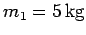, is propelled with speed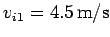toward the second object, mass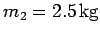, which is initially at rest. After the collision, both objects have velocities which are directed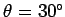on either side of the original line of motion of the first object. What are the final speeds of the two objects? Is the collision elastic or inelastic?Answer: Let us adopt the coordinate system shown in the diagram. Conservation of momentum along the-axis yields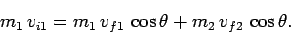Likewise, conservation of momentum along the-axis yields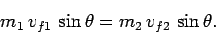The above pair of equations can be combined to give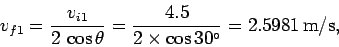and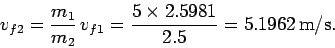The initial kinetic energy of the system is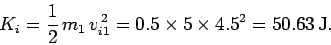The final kinetic energy of the system is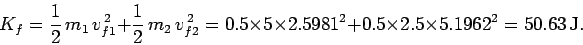Since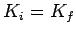, the collision is elastic.Next: Circular motion Up: Conservation of momentum Previous: Worked example 6.5: Elastic
Richard Fitzpatrick 2006-02-02